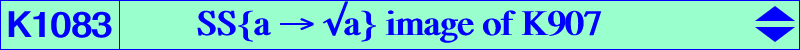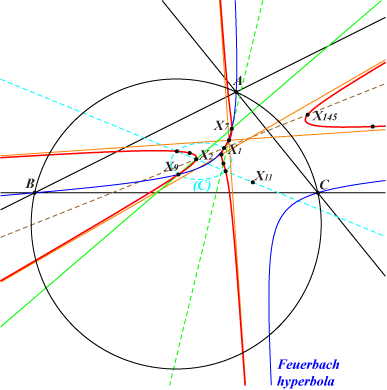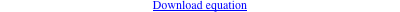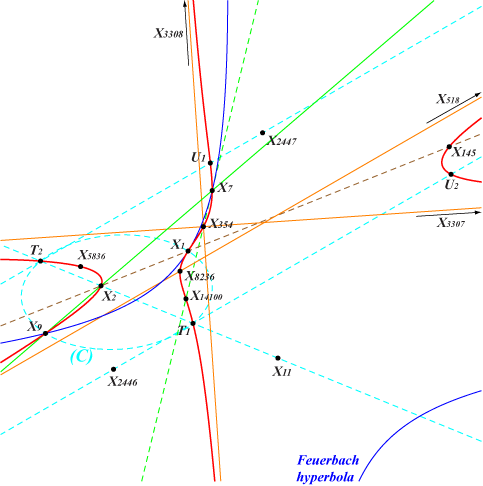too complicated to be written here. Click on the link to download a text file.X(1), X(2), X(7), X(9), X(145), X(354), X(518), X(3158), X(3307), X(3308), X(5836), X(8236), X(14100) see T1, T2, U1, U2, P3, Q3 below Geometric properties :K1083 is the image of the cubic K907 under the symbolic substitution SS{a → √a}. As in Table 62, these two cubics are pKs with respect to a non proper triangle. See the related Q149. All the properties of K907 are easily transposed under SS{a -> √a} and then, let us consider the quadrilateral with vertices X(1), X(9), X(3307), X(3308), the two latter points being the infinite points of the Feuerbach hyperbola. The diagonal triangle of this quadrilateral is a non proper triangle (T) with vertices X(518), T1, T2 where T1, T2 are the common points of the line X(2)X(11) and the conic (C) with center X(1001), passing through X(1), X(9), X(2607), X(9318) which is the SS{a → √a} image of the Brocard circle. These points T1, T2 lie on the parallels to the line X(1)X(6) passing through X(2446), X(2447) respectively. K1083 is then the isogonal pK with pivot X(354), isopivot X(8236) with respect to (T). See the related K1084.This isogonal conjugation in (T) is the mapping that sends a point M to its pole in the pencil of rectangular hyperbolas containing the Feuerbach hyperbola and the diagonal rectangular hyperbola passing through X(1), X(9), X(40), X(188), X(191), X(366), X(1045), X(1050), X(1490), X(2136), X(2949), X(2950), X(2951), X(3174), etc. This hyperbola is the SS{a → √a} image of the Stammler hyperbola. Recall that the Feuerbach hyperbola is the SS{a → √a} image of the Jerabek hyperbola. The isoconjugates U1, U2 of T1, T2 lie on K1083 hence U1 = X(518)X(2447) /\ X(354)T1 and U2 = X(518)X(2446) /\ X(354)T2. *** If the interior of (T) is defined as the region delimited by its sidelines that contains X(1) then X(1) is the incenter of (T) and the circle (C1) with center X(1) is its incircle. The circle (C9) with center X(9) is one of its excircle, the remaining two being decomposed into the line at infinity and the line X(2)X(11) that passes through T1 and T2. In other words, the lines X(1)T1 and X(1)T2 are two internal bisectors of (T) and then the lines X(9)T1 and X(9)T2 are the two corresponding external bisectors of (T). Hence, the two remaining excenters are X(3307), X(3308) on the line at infinity. *** The polar conic of the pivot X(354) is the rectangular hyperbola passing through X(1), X(9), X(3307), X(3308). The polar conic of the isopivot X(8236) is a circum-conic of (T) hence it contains X(518), T1, T2. P3 is the tangential of the isopivot X(8236) hence it is the tertiary pivot of K1083. P3 lies on {1, 971}, {2, 165}. The polar conic of P3 passes through X(2), X(8236), U1, U2. Its isoconjugate is Q3, on the lines {7, 1699}, {165, 518} and obviously on the line X(354)P3.The points above P3, Q3, T1, T2, U1, U2 are now X(24644-5-6-7-8-9) in ETC.